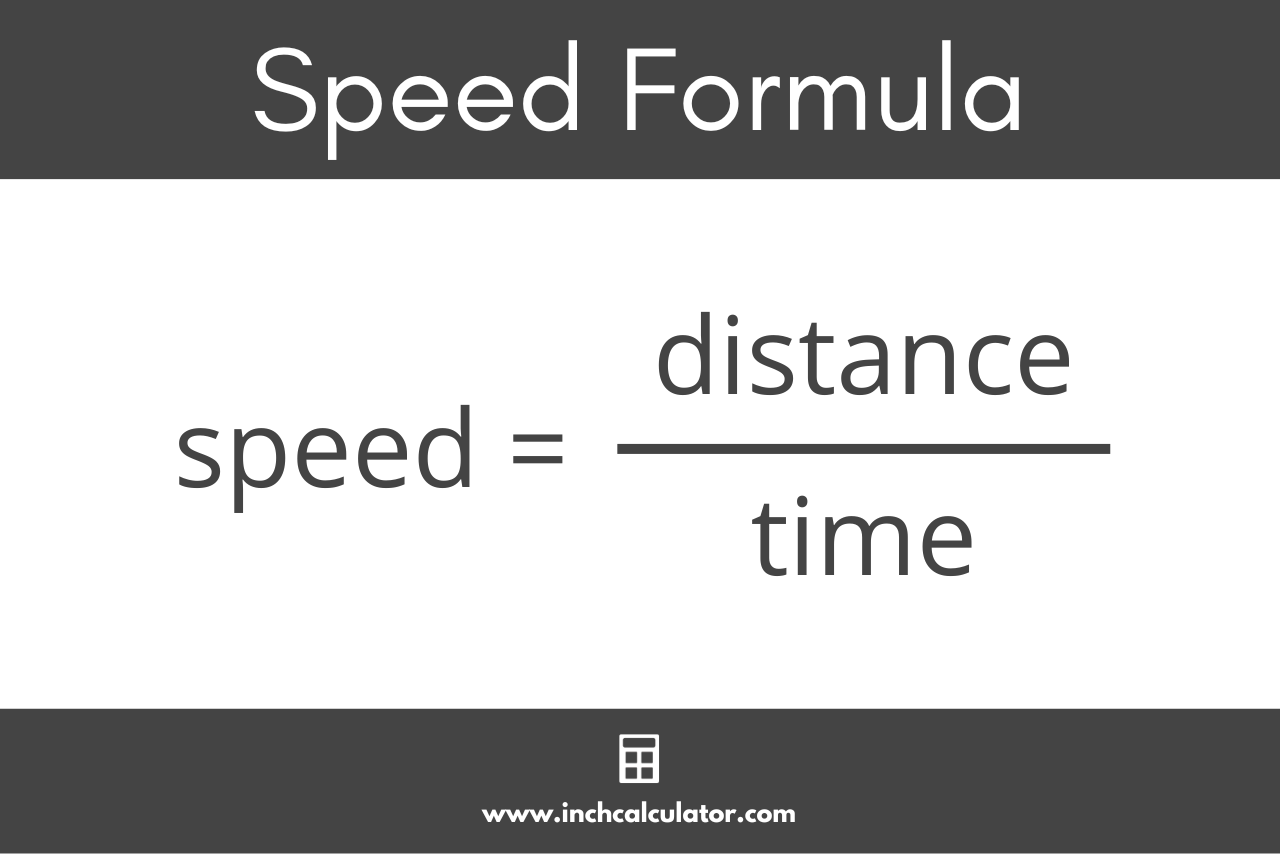# Speed Calculator

Use our speed calculator to calculate the average speed, distance traveled, and the total time duration.

Calculate:
Time:
:
:
Time:
:
:

## Speed:

-
Learn how we calculated this below

## How to Calculate Speed

Speed is a measure of how fast something is moving. It’s the rate at which an object covers distance over time.

You can calculate the average speed using a formula if you know the time and distance.

### Speed Formula

The formula to calculate the speed given the distance an object has traveled and the time it took to travel that distance is:

speed = distance / time

Thus, the average speed is equal to the distance traveled divided by the time duration to cover the distance.It’s important to note that the resulting speed is the average speed over the time duration. It’s possible that the actual speed fluctuates faster and slower during that time; the result of the formula above is the average speed.

For example,

speed = 200 miles / 4 hours
speed = 200 / 4
speed = 50 miles/hour

Thus, the average speed of the car was 50 miles per hour.

### Importance of Units

While calculating speed, it’s crucial to use consistent units.

If the distance is provided in miles and the time in hours, then the speed will be in miles/hour. Similarly, if the distance is given in meters and the time in seconds, the speed will be in meters/second.

If needed, you can use our speed converter to convert one unit of speed to another.

## How to Calculate Distance

Using the speed formula, you can calculate the distance traveled if you know the speed and time duration as follows.

distance = speed × time

The distance traveled is equal to the average speed multiplied by the time duration.

For example,

distance = 6 mph × 2 hours
distance = 6 × 2
distance = 12 miles

In this example, a runner would be able to run 12 miles in 2 hours at a pace of 6 miles per hour.

## How to Calculate Time

Using the speed formula, you can also calculate the duration of time if you know the speed and distance traveled.

time = distance / speed

The duration of time is equal to the distance traveled divided by the average speed. You might also be interested in our time calculator.

For example,

time = 45 miles / 15 mph
time = 45 / 15
time = 3 hours

So, it will take a cyclist 3 hours to ride 45 miles at a speed of 15 mph.

### What is the difference between speed and velocity?

Speed is the rate that an object moves in distance over time, while velocity is the rate that an object changes in position. Velocity is a measure of how fast an object is moving and also which direction it’s moving in.

Speed is a scalar value, measured over time, while velocity is a vector.

### Is speed the same as pace?

No, while speed is the rate that an object moves in distance over time, pace is the average time it takes to cover one mile (or kilometer, meter, foot, etc.). Pace is often used by runners to keep their speed consistent over the course of a race or run.

Pace is often used rather than speed for running and cycling since it allows measuring the time it takes to cover a set distance. You can learn more using our pace calculator.

### What are common speed units?

The most common units of speed are:

• kilometers per hour (km/h)
• miles per hour (mph)
• meters per second (m/s)
• feet per second (ft/s)